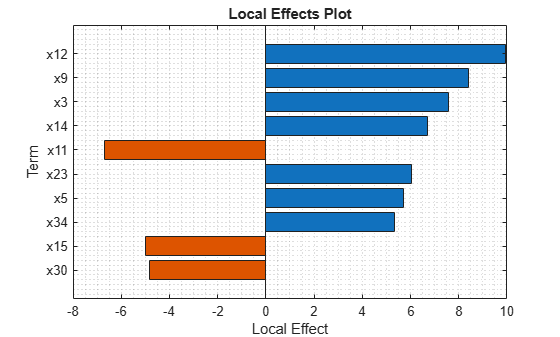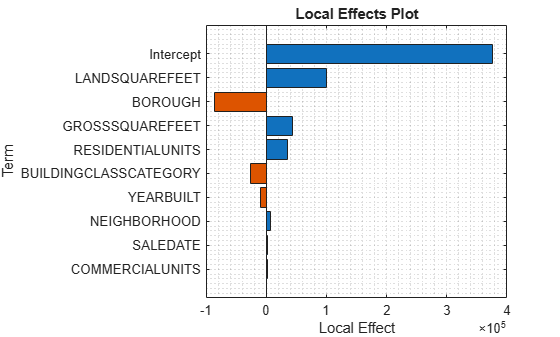# plotLocalEffects

Plot local effects of terms in generalized additive model (GAM)

Since R2021a

## Syntax

``plotLocalEffects(Mdl,queryPoint)``
``plotLocalEffects(Mdl,queryPoint,Name,Value)``
``plotLocalEffects(___)``

## Description

example

````plotLocalEffects(Mdl,queryPoint)` creates a bar graph showing the local effects of the terms in the generalized additive model `Mdl` on the prediction at the specified query point `queryPoint`.```
````plotLocalEffects(Mdl,queryPoint,Name,Value)` specifies additional options using one or more name-value arguments. For example, `'IncludeIntercept',true` specifies to include an intercept term in the bar graph.```

example

````b` = `plotLocalEffects(___)` returns a bar graph object `b` using any of the input argument combinations in the previous syntaxes. Use `b` to query or modify Bar Properties of the bar graph after it is created. ```

## Examples

collapse all

Train a univariate generalized additive classification model, which contains linear terms for predictors. Classify a new observation using a memory-efficient model object. Then, interpret the prediction for a specified data instance by using the `plotLocalEffects` function.

Load the `ionosphere` data set. This data set has 34 predictors and 351 binary responses for radar returns, either bad (`'b'`) or good (`'g'`).

`load ionosphere`

Train a univariate GAM that identifies whether the radar return is bad (`'b'`) or good (`'g'`).

`Mdl = fitcgam(X,Y);`

`Mdl` is a `ClassificationGAM` model object.

Conserve memory by reducing the size of the trained model.

`CMdl = compact(Mdl);`

Classify the first observation of the training data, and plot the local effects of the terms in `Mdl` on the prediction.

`label = predict(CMdl,X(1,:))`
```label = 1x1 cell array {'g'} ```
`plotLocalEffects(CMdl,X(1,:))`The `predict` function classifies the first observation `X(1,:)` as `'g'`. The `plotLocalEffects` function creates a horizontal bar graph that shows the local effects of the 10 most important terms on the prediction. Each local effect value shows the contribution of each term to the classification score for `'g'`, which is the logit of the posterior probability that the classification is `'g'` for the observation.

Train a GAM for binary classification with both linear and interaction terms for predictors. Create local effects plot using both linear and interaction terms in the model, and then create a plot using only linear terms in the model. Specify whether to include interaction terms when creating the local effects plot.

Load the `ionosphere` data set. This data set has 34 predictors and 351 binary responses for radar returns, either bad (`'b'`) or good (`'g'`).

`load ionosphere`

Train a GAM using the predictors `X` and class labels `Y`. A recommended practice is to specify the class names. Specify to include the 10 most important interaction terms.

`Mdl = fitcgam(X,Y,'ClassNames',{'b','g'},'Interactions',10);`

`Mdl` is a `ClassificationGAM` model object.

Create local effects plots for the 10th observation. Use both the linear and interaction terms in `Mdl` for the first plot, and use only the linear terms in `Mdl` for the second plot. To exclude interaction terms, specify `'IncludeInteractions',false`.

```t = tiledlayout(2,1); title(t,'Local Effects Plots for 10th Observation') nexttile plotLocalEffects(Mdl,X(10,:)) title('GAM with linear and interaction terms') nexttile plotLocalEffects(Mdl,X(10,:),'IncludeInteractions',false) title('GAM with only linear terms')```The plots display the 10 most important terms. Both plots include nine common terms and one uncommon term. The first plot includes the interaction term for `x1` and `x5`, whereas the second plot includes the linear term for `x14`.

Train a univariate GAM for regression, which contains linear terms for predictors. Then, interpret the prediction for a specified data instance by using the `plotLocalEffects` function.

Load the data set `NYCHousing2015`.

`load NYCHousing2015`

The data set includes 10 variables with information on the sales of properties in New York City in 2015. This example uses these variables to analyze the sale prices (`SALEPRICE`).

Preprocess the data set. Remove outliers, convert the `datetime` array (`SALEDATE`) to the month numbers, and move the response variable (`SALEPRICE`) to the last column.

```idx = isoutlier(NYCHousing2015.SALEPRICE); NYCHousing2015(idx,:) = []; NYCHousing2015.SALEDATE = month(NYCHousing2015.SALEDATE); NYCHousing2015 = movevars(NYCHousing2015,'SALEPRICE','After','SALEDATE');```

Display the first three rows of the table.

`head(NYCHousing2015,3)`
``` BOROUGH NEIGHBORHOOD BUILDINGCLASSCATEGORY RESIDENTIALUNITS COMMERCIALUNITS LANDSQUAREFEET GROSSSQUAREFEET YEARBUILT SALEDATE SALEPRICE _______ ____________ ____________________________ ________________ _______________ ______________ _______________ _________ ________ _________ 2 {'BATHGATE'} {'01 ONE FAMILY DWELLINGS'} 1 0 4750 2619 1899 8 0 2 {'BATHGATE'} {'01 ONE FAMILY DWELLINGS'} 1 0 4750 2619 1899 8 0 2 {'BATHGATE'} {'01 ONE FAMILY DWELLINGS'} 1 1 1287 2528 1899 12 0 ```

Train a univariate GAM for the sale prices. Specify the variables for `BOROUGH`, `NEIGHBORHOOD`, `BUILDINGCLASSCATEGORY`, and `SALEDATE` as categorical predictors.

`Mdl = fitrgam(NYCHousing2015,'SALEPRICE','CategoricalPredictors',[1 2 3 9]);`

`Mdl` is a `RegressionGAM` model object.

Display the estimated intercept (constant) term of `Mdl`.

`Mdl.Intercept`
```ans = 3.7518e+05 ```

The intercept term value is close to the average of the response variable in a regression GAM if the training data does not include `NaN` values. Compute average of the response variable.

`mean(NYCHousing2015.SALEPRICE)`
```ans = 3.7518e+05 ```

Predict the sale price for the first observation of the training data, and plot the local effects of the terms in `Mdl` on the prediction. Specify `'IncludeIntercept',true` to include the intercept term in the plot.

`yFit = predict(Mdl,NYCHousing2015(1,:))`
```yFit = 4.4421e+05 ```
`plotLocalEffects(Mdl,NYCHousing2015(1,:),'IncludeIntercept',true)`The `predict` function predicts the sale price for the first observation as `4.4421e5`. The `plotLocalEffects` function creates a horizontal bar graph that shows the local effects of the terms in `Mdl` on the prediction. Each local effect value shows the contribution of each term to the predicted sale price.

## Input Arguments

collapse all

Query point at which `plotLocalEffects` plots the local effects, specified as a row vector of numeric values or a single-row table.

• For a row vector of numeric values:

• The variables that makes up the columns of `queryPoint` must have the same order as the predictor variables that trained `Mdl`.

• If you trained `Mdl` using a table (for example, `Tbl`), then `queryPoint` can be a numeric matrix if `Tbl` contains all numeric variables.

• For a single-row table:

• If you trained `Mdl` using a table (for example, `Tbl`), then all predictor variables in `queryPoint` must have the same variable names and data types as those in `Tbl`. However, the column order of `queryPoint` does not need to correspond to the column order of `Tbl`.

• If you trained `Mdl` using a numeric matrix, then the predictor names in `Mdl.PredictorNames` and the corresponding predictor variable names in `queryPoint` must be the same. To specify predictor names during training, use the `'PredictorNames'` name-value argument. All predictor variables in `queryPoint` must be numeric vectors.

• `queryPoint` can contain additional variables (response variables, observation weights, and so on), but `plotLocalEffects` ignores them.

• `plotLocalEffects` does not support multicolumn variables or cell arrays other than cell arrays of character vectors.

Data Types: `single` | `double` | `table`

### Name-Value Arguments

Specify optional pairs of arguments as `Name1=Value1,...,NameN=ValueN`, where `Name` is the argument name and `Value` is the corresponding value. Name-value arguments must appear after other arguments, but the order of the pairs does not matter.

Before R2021a, use commas to separate each name and value, and enclose `Name` in quotes.

Example: `plotLocalEffects(Mdl,queryPoint,'IncludeInteractions',false,'NumTerms',5)` specifies to create a bar plot containing the five most important linear terms for predictors in `Mdl` excluding the interaction terms in `Mdl`.

Flag to include interaction terms of the model in the plot, specified as `true` or `false`.

The default `'IncludeInteractions'` value is `true` if `Mdl` contains interaction terms. The value must be `false` if the model does not contain interaction terms.

Example: `'IncludeInteractions',false`

Data Types: `logical`

Flag to include an intercept term of the model in the plot, specified as `true` or `false`.

Example: `'IncludeIntercept',true`

Data Types: `logical`

Number of terms to plot, specified as a positive integer scalar. `plotLocalEffects` plots the specified number of terms with the highest absolute local effect values.

Example: `'NumTerms',5` specifies to plot the five most important terms. `plotLocalEffects` determines the order of importance by using the absolute local effect values.

Data Types: `single` | `double`

## Version History

Introduced in R2021a